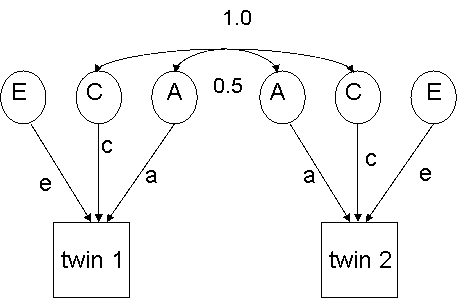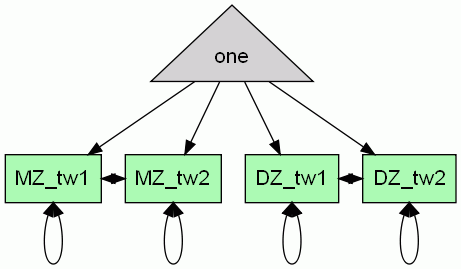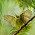## Sunday, 28 August 2011

### SEM approach to twin data

For behavior genetic analysis, our interest is in how far phenotypic similarities between individuals can be accounted for in terms of their degree of genetic similarity. In the simplest kind of twin analysis, our observed data consists of two covariance matrices, one for MZ (identical) and one for DZ (fraternal twins), representing the covariance on a single measured trait between two members of a twin pair. The key assumption behind the twin method is that similarities and differences between pairs of individuals can be expressed in terms of genes, their common environment (which serves to make twins resemble one another) and their specific (nonshared) environment (which makes twins different from one another). One point that can cause confusion is when we talk of genetic similarity between individuals: it is common to find a statement that MZ twins have all their genes in common, whereas DZ twins have 50% in common. This is puzzling when we also hear that humans have 98% of their genes in common with chimpanzees! The point is that in behavior genetics we are only interested in genes that take different allelic forms in different people, and so can potentially account for individual differences. These polymorphic (or 'segregating') genes are a very small proportion of the human genome.
If we focus for the time being just on additive genetic influences, then the relationship between a set of DZ twins can be depicted in the standard ACE model,as shown in figure 7.Figure 7: Standard ACE model: DZ twins

Each latent factor has its associated path, and it is the goal of a behavior genetic analysis to estimate these paths. Note that the same letters are used for paths from latent factors to twin 1 and twin 2, indicating that in our model we assume no differences in the size of genetic and environmental influences for the two twins. Provided twins are assigned as twin 1 and twin 2 at random, this is a valid assumption.

Note that the path between the two latent C factors (common environment) is set to 1, as this is, by definition, identical for twin 1 and twin 2. The path between the latent A factors is set to .5, reflecting the fact that DZ twins share 50% of their segregating alleles on average.

Path-tracing rules can be applied to estimate the expected covariance between twin 1 and 2:
CovDZ = .5 * a2 + c2
Also, the variance for scores of either set of twins can be estimated:
VarDZ  = a2 + c2+ e2
For MZ twins,  the model is virtually identical, except that the correlation between the A terms is set to 1.0, because it is assumed that MZ twins have the same alleles for all genes. Therefore, the estimates of variance for MZ twins is the same as for DZ twins, but the estimates of covariance between twin 1 and twin 2 is now:
CovMZ =  a2 + c2
If we used standardized variables, then the total variance for each twin will be one, and a2 will be a direct measure of the proportion of variance that can be accounted for in terms of additive genetic influences, i.e. heritability. The formulae from path-tracing indicate that we can estimate the size of a2, c2 and e2 just from a knowledge of the correlations between twin 1 and twin 2 in DZ and MZ pairs. Because correlations are standardized, we will get the estimates in standard units representing proportion of variance accounted for.
Thus simple algebra allows us to estimate:
(CorMZ-CorDZ) = .5 *  a2
so
a2 = 2 *(CorMZ-CorDZ)
c2 = CorMZ - a2
e2 = 1 - a2 - c2
You might start to wonder why we should do complicated model-fitting when it is easy to get these estimates just from a couple of correlations!  There are three reasons. First, model-fitting provides a more stringent test, because it will be sensitive to any violations of the assumptions of the model. Model-fitting usually estimates covariances rather than correlations, so that we can confirm, for instance, that the variances are comparable for MZ and DZ twin pairs. The model assumes they are the same, and so the fit of the model will be reduced if this assumption is not met.  Second, with model-fitting we can not only estimate the paths, but also obtain confidence intervals around our estimates, and compare the fit of nested models, as will be illustrated more fully below. Third, with a model-fitting approach, we can easily scale up models to account for multivariate data, as well as formulating more complex models that include, for instance, other variables, measured environmental influences, or specific allelic effects.

Modeling twin data in OpenMx
As we shall see, there are two approaches to modeling twin data in OpenMx. The first uses a matrix algebra representation; the second uses the RAM path method that was illustrated above. Both will be illustrated here and readers can decide which approach they prefer. For beginners, it can also be useful to specify a model using both methods to check that they give the same results. Note that the omxGraphviz command for creating a diagram works only with RAM type models.
Traditional approaches to twin modeling used covariance matrices for DZ and MZ twin pairs as input to the model, but more recently it has become standard to have raw data as the input. This can make data input easier (since the original data can be used) and also allows us to include cases with missing data. We will see that with OpenMx models using the FIML optimization approach, we are required to provide estimates for means as well as covariances.

For the next example, we will use simulated data on twins. Instructions for how to read in your own data will be given after we have gone through the ACE model, so you can try it out with real life data.
The following script simulates data on 1000 pairs of MZ and DZ twins, assuming a2 of .5, c2 of .3 and e2 of .2.
#----------------------------------------------------------------------------------------------------------------------
#                                                             Twin simulate
# DVM Bishop, 11th March 2010, Based on script in OpenMXUserGuide, p 15
#----------------------------------------------------------------------------------------------------------------------
require(OpenMx)   # not needed for the simulation, but will be needed when we come to model specification
require(MASS)    # needed for multivariate random number generation
set.seed(200)        # specified seed ensures same random number set generated on each run

mya2<-0.5 #Additive genetic variance component (a squared)
myc2<-0.3 #Common environment variance component (c squared)
mye2<-1-mya2-myc2 #Specific environment variance component (e squared)

my_rMZ <-mya2+myc2          # correlation between MZ twin1 and twin2
my_rDZ <- .5*mya2+myc2     # correlation between DZ twin 1 and twin 2

myDataMZ <- mvrnorm (1000, c(0,0), matrix(c(1,my_rMZ,my_rMZ,1),2,2))
myDataDZ <- mvrnorm (1000, c(0,0), matrix(c(1,my_rDZ,my_rDZ,1),2,2))

colnames(myDataMZ) <- c('twin1', 'twin2') # assign column names
summary(myDataMZ)
colMeans(myDataMZ,na.rm=TRUE)
#na.rm means ignore NA values (non-numeric)
cov(myDataMZ,use="complete")
# "complete" specifies use only cases with data in all columns

# do scatterplots for MZ and DZ
split.screen(c(1,2))        # split display into two screens side by side
# (use c(2,1) for screens one above the other)
screen(1)
plot(myDataMZ,main='MZ')    # main specifies overall plot title
screen(2)
#use drag and drop to resize the plot window if necessary

colnames(alltwin)=c("MZ_twin1","MZ_twin2","DZ_twin1","DZ_twin2")
write.table(alltwin,"mytwinfile")
# Saves a copy of mydata in your R directory under name "mytwinfile"
#--------------------------------------------------------------------------------------------

Notice that although we have no missing data in our simulated file, the commands above are specified to allow for missing data. Create a copy of myDataDZ with the command:
and in the editor, type NA over one of the entries in each column. NA is the R code for a missing value. Now type:
cov(nudat)
colMeans(nudat)

You will see that the results just give NA values. However, the missing values are ignored if you type:

We start by fitting a saturated model, estimating means, variances and covariances separately for each group of twins (twin 1 vs twin 2) and by zygosity (MZ vs DZ pairs), so we can get a baseline likelihood estimate against which to compare a genetic model . The saturated model will have two matrices for expected means (one each for MZs and DZs), and two for the expected covariances, generated from multiplying a lower triangular matrix with its transpose (i.e. the Cholesky decomposition). The raw data to be used are specified using the mxData command. The function for optimization is mxFIMLObjective applied. We start with the submodel for MZ twins, as follows:

#--------------------------------------------------------------------------------------------
#  MZ submodel
#--------------------------------------------------------------------------------------------
mxModel("MZ",
mxMatrix(
type="Full",
nrow=1,
ncol=2,
free=TRUE,
values=c(0,0),
name="expMeanMZ"),
mxMatrix(
type="Lower",
nrow=2,
ncol=2,
free=TRUE,
values=.5,
name="CholMZ"),
mxAlgebra(
CholMZ %*% t(CholMZ),
name="expCovMZ"),
mxData(
myDataMZ,
type="raw"),
mxFIMLObjective("expCovMZ", "expMeanMZ"))
#--------------------------------------------------------------------------------------------

Note that  all subcommands within a model must end with a comma,  until we get to the last subcommand, which terminates the model with a final close bracket. We will now illustrate a closely parallel model for the DZ twins, but will take the opportunity to say a word about formatting.

Formatting OpenMx commands
You may encounter scripts that use a more compact form, as illustrated below for the DZ group. Note that this script is formally identical to that for MZ twins, except for the 'name' specifications.  If the arguments to the OpenMx command are given in the default order  ( see help(mxMatrix) to go to the help/reference page which lists the arguments)  then it is not necessary to include the name of the argument. The script has skipped a few optional arguments, so the argument "name=" has to be explicitly provided to refer to the last argument. In general, we would not recommend this compact format, as it is hard for other users to understand. The formatting used for the MZ group above, with soft tabs and each argument on a separate line is much clearer.

#--------------------------------------------------------------------------------------------
#  DZ submodel, illustrating compact formatting
#--------------------------------------------------------------------------------------------
mxModel("DZ",
mxMatrix("Full", 1, 2, T, c(0,0), name="expMeanDZ"),
mxMatrix("Lower", 2, 2, T, .5, name="CholDZ"),
mxAlgebra(CholDZ %*% t(CholDZ), name="expCovDZ"),
mxFIMLObjective("expCovDZ", "expMeanDZ", mylabels))
#--------------------------------------------------------------------------------------------
Combining models into a supermodel
The MZ and DZ models are now combined in a 'super' model, which includes them as arguments. To obtain the overall likelihood of data for MZ and DZ twins, we simply add together the log likelihoods for MZ and DZ submodels. The super-model includes an mxAlgebra statement to do this. This is given the name 'twin'.
To evaluate the models simultaneously, we use the mxAlgebraObjective with 'twin' as its argument.

Before we fit the ACE model, we will fit the 'saturated' model, which ignores the  relationships between twin pairs. In the script below, the models for MZ and DZ pairs are identical. This acts as a baseline against which the ACE model can be assessed. It has no latent variables, just observed means and covariances.

#---------------------------------------------------------------------------------------------
#            Saturated_twin_model
#            DVM Bishop, 12th March 2010, based on OpenMxUsersGuide, p. 16
#---------------------------------------------------------------------------------------------
require(OpenMx)
#read in previously saved data created with Twin Simulate script
myDataMZ=mytwindata[,1:2]  #columns 1-2 are MZ twin1 and twin2
myDataDZ=mytwindata[,3:4]  #columns 3-4 are DZ twin 1 and twin 2
colnames(myDataMZ)=c("twin1","twin2")
mylabels=c("twin1","twin2")

# Model specification starts here
mytwinSatModel <- mxModel("twinSat",
mxModel("MZ",
mxMatrix(type="Full",
nrow=1,
ncol= 2,
free=TRUE,
values=c(0,0),
name="expMeanMZ"),
mxMatrix("Lower",
nrow= 2,
ncol=2,
free=TRUE,
values=.5,
name="CholMZ"),
mxAlgebra(CholMZ %*% t(CholMZ), name="expCovMZ"),
mxData(myDataMZ, type="raw"),
mxFIMLObjective("expCovMZ", "expMeanMZ", mylabels)),

mxModel("DZ",
mxMatrix(type="Full",
nrow=1,
ncol= 2,
free=TRUE,
values=c(0,0),
name="expMeanDZ"),
mxMatrix(type="Lower",
nrow=2,
ncol=2,
free=TRUE,
values=.5,
name="CholDZ"),
mxAlgebra(CholDZ %*% t(CholDZ), name="expCovDZ"),
mxFIMLObjective("expCovDZ", "expMeanDZ", mylabels)),

mxAlgebra(MZ.objective + DZ.objective, name="twin"),
# adds together likelihoods for MZ and DZ groups
mxAlgebraObjective("twin"))
# evaluate expression from mxAlgebra, i.e. both submodels together
#---------------------------------------------------------------------------------------------------------------------------
mytwinSatFit <- mxRun(mytwinSatModel) #The mxRun command evaluates the model.
myExpMeanMZ <- mxEval(MZ.expMeanMZ, mytwinSatFit)
# you can type the name of any of these assigned variables to see the results
myExpCovMZ <- mxEval(MZ.expCovMZ, mytwinSatFit)
myExpMeanDZ <- mxEval(DZ.expMeanDZ, mytwinSatFit)
myExpCovDZ <- mxEval(DZ.expCovDZ, mytwinSatFit)
LL_Sat <- mxEval(objective, mytwinSatFit)
summary(mxRun(mytwinSatModel))
#--------------------------------------------------------------------------------------------------------------------------
# compute DF for this model - this is a clunky way to do it!
msize=nrow(myDataMZ)*ncol(myDataMZ)
myDF_Sat=msize+dsize-nrow(mytwinSatFit@output\$standardErrors)
#-------------------------------------------------------------------------------------------------------------------------
Study the summary output and make sure you understand it. Note that when fitting a model to raw data, the degrees of freedom will reflect the sample size, being equivalent to the total number of observations, minus the estimated parameters. In this case, the total number of observations is 4000 (2 observations for 1000 MZ and 1000 DZ pairs),  and the N estimated parameters is 10, so the DF is 3990.
• Can you draw a path diagram of the saturated model? (NB we can't generate a diagram with omxGraphviz because we are not using a RAM model).
• What are the ten estimated parameters?
• Can you work out how you would compute the estimated DZ covariance matrix from the three DZ.CholDZ values given in rows 8-10 of the output, just using R commands?
• Do you understand why chi square is designated as NA, rather than given a numerical value?
One way to computed the estimated DZ covariance would be to copy the three values from DZ.CholDZ into a 2 x 2 matrix, with zero in the upper right-hand entry, thus:
a=matrix(c(.9968,.5562,0,.80269),nrow=2)
and then multiply a by its transpose. You should check how the result compares with the covariance matrix you get if you type:

Figure 8 shows you the path diagram for the saturated model. The four paths from 'one' to the four boxes correspond to the four estimated means, the looped two-headed arrows attached to each box correspond to variances, and the two-headed arrows linking twins of the same zygosity correspond to covariances. Note that there is one arrow for each observed covariance and variance, so the degrees of freedom are zero. There are no latent variables, just observed variables (in boxes) and a constant (in triangle).Figure 8: Path Diagram of Saturated Model

Before we move on to fit the ACE model to the same data, we will test some of the assumptions of the twin model, i.e. that the means and variances are the same for twin 1 and twin 2, and that they are the same for MZ and DZ twins.

Equating parameters by giving them the same label
A key feature of OpenMx is that if two estimated parameters have the same label, then they will be treated as identical. To demonstrate this point, return to the previous script, and insert the following line in the sections where the matrices for expMeanMZ and expMeanDZ are defined, immediately above the 'name' statement for each matrix:
labels="mean",
Before you re-run the script, make a note of the -2LL values and DF from the original script,  and think about what this change will do. We have given all four means the same label, so they will be estimated to all have the same value. How will this affect the degrees of freedom?  Now run the modified script and again check the -2LL values and the DF.

You should get a value of 9911.62 for the original script, with 3990 degrees of freedom, and 9913.154 for the script with equated means, and 3993 degrees of freedom. You should be able to use this information to determine whether  the means in this sample do depart significantly from the expectation that they will all have the same value.

#### 1 comment:

1.This comment has been removed by a blog administrator.